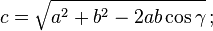This weekend I was wondering if it is possible to use triangulation to pinpoint the position of an object using two ultrasonic sensors. So I made a simple robot and wrote a small program to find out. It proves this can be done.Triangulation is a technique that uses angles or distances between points to calculate the position of these points. GPS localization for example uses triangulation. The technique is based on the law of cosines. This law allows you to calculate the angles of a triangle given the lengths of its sides. But also to calculate the length of one side given the length of the two other sides and the angle of the opposite corner. This might sound complicated, but they teach it in high school, so you might know it.

This means that a robot equipped with two US sensors can calculate the exact position of an object, provided it is in sight of these two sensors. Potentially, this could be a huge benefit. Because a single sensor has a wide (30 degrees) detection beam, giving much uncertainty about the exact location of an object. But a single sensor also deforms the apparent shape of an object. Practically this means that constructing maps using a single US sensor is impossible. Maybe, with two sensors and some triangulation a map can be constructed, as the position of the objects can be calculated.Here is a picture of the setup I used to test if it is practically possible to do this with two Lego US sensors. The sensors can be rotated as they are mounted on the shaft of a NXT motor. The sensors are constantly pointing to the object in sight. If no object is in sight the sensors point straight ahead, waiting for an object. It is clear to see from the rotation of the sensors where they are “looking” at. The effectiveness of the setup is clear to everyone.

After some tests I am pleased with the effectiveness of this setup. It is accurate up to 2-4 cm. If this is enough to produce good maps I don’t know. But it is at least effective for a whole range of other tasks. I’m thinking of shooting cannons, search missions and object avoidance. The video gives you an impression.The effective range of the setup is limited as the picture to the right shows. I put a piece of Lego on every spot I got a successful hit. (The eleven hole beam is there for reference). It is not as wide or long as you might think. I think there are two reasons for that. the main reason is because of the low resolution of the sensor,  just one cm. This causes numerical errors whose effects are  small along the forward pointing axis, but that get larger to the sides. Offset error of the sensors is the second cause that limit the sideways effectiveness. Scale errors of the sensors limit the length of the effective range.

You might wonder how good a rigid setup works. Then the sensors are not allowed to rotate and home in on the object. Well, obviously the field of view is much smaller, and the sensors should be placed facing inward to maximize field of view. But I noticed that the result is less accurate and stable.

The program I wrote is very simple. It is just these two forms of the law of cosines embedded in some control structures.```package lejos.nxt.sensor.example;

import lejos.nxt.Button;
import lejos.nxt.LCD;
import lejos.nxt.Motor;
import lejos.nxt.NXTRegulatedMotor;
import lejos.nxt.SensorPort;
import lejos.nxt.sensor.sensor.LcUltrasonic;
import lejos.util.Delay;

public class TwoUSSensors {

static final int P=5;							// P component of the P-controller
static final double MARGIN=0.8;		// Sets the margin of max distance for two measurements to assume a single object in sight
static final float MAXRANGE=150;	// sets the macimum range of the sensor
static final int MINANGLE=25;			// sets the minimum angle a sensor can rotate to(o means the two sensors are facing each other
static final double b=20.5;				// sets the distance between the two sensors, measured from shaft to shaft
static final float SENSOROFFSET=2.5f;

static final LcUltrasonic sensorA=new LcUltrasonic(SensorPort.S1);
static final LcUltrasonic sensorC=new LcUltrasonic(SensorPort.S4);

static final NXTRegulatedMotor C=Motor.C;
static final NXTRegulatedMotor A=Motor.A;

public static void main(String[] args) {

Object run=new TwoUSSensors();

}

public TwoUSSensors() {
double targetC=0, targetA=0;
double  a,c;
sensorA.setMode(LcUltrasonic.MODE_PING);
sensorC.setMode(LcUltrasonic.MODE_PING);

C.rotateTo(90, true);
A.rotateTo(90, false);
Delay.msDelay(500);

while(!Button.ESCAPE.isDown()) {

c=corrrectedA.fetchSample();
a=corrrectedA.fetchSample();

LCD.clear();
LCD.drawString("       A     C", 0, 0);
LCD.drawString("dist", 0, 1);
LCD.drawString("dist'", 0, 2);
LCD.drawString("target", 0, 3);
LCD.drawString("error", 0, 4);
LCD.drawString("state", 0, 5);

LCD.drawInt((int) c, 7, 1);
LCD.drawInt((int) a, 12, 1);

if (a>MAXRANGE && c> MAXRANGE) {
// it is assumed that there are no objects in sight
targetA = Math.PI/2;
targetC = Math.PI/2;
LCD.drawString("-", 7, 5);
LCD.drawString("-", 12, 5);
}
else {
// it is assumed there are objects in sight
if (Math.abs(a-c)>b*MARGIN) {
// it is assumed that there are two different objects in sight
if (a<c) {
// it is assumed there is an object in front of sensorC
LCD.drawString("-", 7, 5);
LCD.drawString("+", 12, 5);
c=Math.sqrt(a*a+b*b-2*a*b*Math.cos(targetC)); // the distance between sensorA and object;
targetA =	Math.acos((a*a-b*b-c*c)/(-2*c*b));
}
else {
// it is assumed there is an object in front of sensorA
LCD.drawString("+", 7, 5);
LCD.drawString("-", 12, 5);
a=Math.sqrt(b*b+c*c-2*b*c*Math.cos(targetA)); // the distance between sensorA and object;
targetC =Math.acos((c*c-a*a-b*b)/(-2*a*b));
}
LCD.drawInt((int) c, 7, 2);
LCD.drawInt((int) a, 12, 2);
}
else {
LCD.drawString("+", 7, 5);
LCD.drawString("+", 12, 5);
// it is assumed that there is one object in sight
targetC =Math.acos((c*c-a*a-b*b)/(-2*a*b));
targetA =Math.acos((a*a-b*b-c*c)/(-2*c*b));
}
}

LCD.drawInt((int) Math.toDegrees(targetA),7,3);
LCD.drawInt((int) Math.toDegrees(targetC),12,3);

// set speed
LCD.drawInt(rotateTo(A, targetA),7,4);
LCD.drawInt(rotateTo(C, targetC),12,4);
Delay.msDelay(20);
}

A.setSpeed(200);
C.setSpeed(200);
C.rotateTo(0, true);
A.rotateTo(0, false);
Delay.msDelay(500);
}

int rotateTo(NXTRegulatedMotor motor,double target){
int targetDeg=(int) Math.toDegrees(target);

if (targetDeg<MINANGLE) target=MINANGLE;
int error=targetDeg-motor.getPosition();
if (Math.abs(error)<=1) return error;
motor.setSpeed(error*P);
motor.rotateTo(targetDeg, true);
return error;
}

}

```